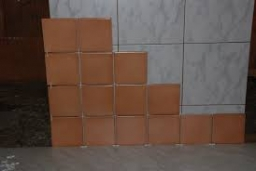# Together 8086

The first tiler would cover the inside of the pool in 5 working days, and the second would need seven working days for the same work.

In how many days would they line the inside of the pool together?

t =  2.9167 d

### Step-by-step explanation:

t·(1/5+1/7)=1

12t = 35

t = 35/12 = 2.91666667

t = 35/12 ≈ 2.916667

Our simple equation calculator calculates it.Did you find an error or inaccuracy? Feel free to write us. Thank you!

Tips for related online calculators
Do you have a linear equation or system of equations and looking for its solution? Or do you have a quadratic equation?
Do you want to convert time units like minutes to seconds?